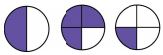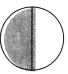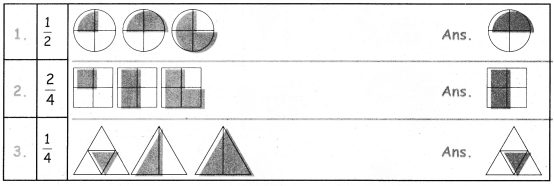Tamilnadu State Board New Syllabus Samacheer Kalvi 4th Maths Guide Pdf Term 3 Chapter 6 Fraction Ex 6.5 Textbook Questions and Answers, Notes.

## Tamilnadu Samacheer Kalvi 4th Maths Solutions Term 3 Chapter 6 Fraction Ex 6.5

I. Choose the appropriate picture given below for the fraction $$\frac { 2 }{ 4 }$$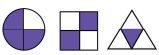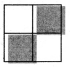Hint: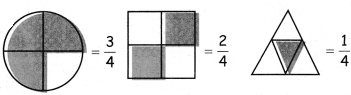II. Choose the appropriate picture given below for the fraction $$\frac { 1 }{ 2 }$$